# Numbers - math word problems

#### Number of problems found: 1462

• Bikes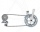Pepa asked Honza how many bikes he had. He replied that he had three-quarters of their total number and another three-quarter of one bike. So how many bikes does Honza have?
• RainfallAnnual rainfall in our country is an average of 797 mm. How many m3 of water rains on average per hectare?
• Reminder and quotientThere are given the number C = 281, D = 201. Find the highest natural number S so that the C:S, D:S are with the remainder of 1,
• Tourist cottage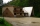The tourist cottage has 11 rooms for 16 guests, some of them are doubles, some singles. How many are double rooms and how many are single rooms?
• 600 pencils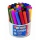600 pencils we want to be divided into three groups. The biggest groups have ten pens more than the smallest. How many ways can this be done?
• Voting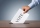400 employees cast their votes in a board member election that has only 2 candidates. 120 people vote for candidate A, while half of the remaining voters abstain. How many votes does candidate B receive?
• Candy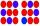How many ways can divide 10 identical candies to 5 children?
• Four integersFnd four consecutive integers so that the product of the first two is 70 times smaller than the product of the next two.
• The King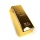The King wants to divide his sons equally. He has 42 rubies and 45 diamonds. How many sons and how will they share them?
• Homework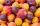In the crate are 18 plums, 27 apricot and 36 nuts. How many pieces of fruit left in the crate when Peter took 8 ninth: 1. nuts 2. apricots 3. fruit 4. drupeProduct of two numbers is 900. If we increase lowest number by 2 then product will increase by 150. Determine both numbers.On the meadow grazing horses, cows, and sheep, together with less than 200. If cows were 45 times more, horses 60 times more, and sheep 35 times more than there are now, their numbers would equally. How many horses, cows, and sheep are on the meadow toget
• Twelve flowers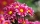A florist has roses, tulips, daffodils, and carnations to use in flower arrangements. If she were to make an arrangement using 12 flowers, how many different combinations of these 4 types of flowers would be possible?
• Two numbersWe have two numbers. Their sum is 140. One-fifth of the first number is equal to half the second number. Determine those unknown numbers.
• One hundred stamps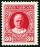A hundred letter stamps cost a hundred crowns. Its costs are four levels - twenty tenths , one crown, two-crown and five-crown. How many are each type of stamps? How many does the problem have solutions?
• LunchSeven classmates go every day for lunch. If they always come to the front in a different order, will be enough school year to take of all the possibilities?
• IS triangleCalculate interior angles of the isosceles triangle with base 40 cm and legs 22 cm long.
• LibraryNew books were purchased for the library. Five-eighths were professional books, one-fifth were encyclopedias, and 231 books were dictionaries. How many professional books were there?
• Peter and PaulPeter and Paul together have 26 years. Four years ago, Paul was twice older than Peter. How much is Paul and how much Peter?
• Grandma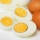Grandma bought two 10 pack of eggs. She used 8 eggs on the cake, 2 times less on the omelette and 4 more eggs for breakfast. How many eggs did it stand for?

Do you have an interesting mathematical word problem that you can't solve it? Submit a math problem, and we can try to solve it.

We will send a solution to your e-mail address. Solved examples are also published here. Please enter the e-mail correctly and check whether you don't have a full mailbox.

Please do not submit problems from current active competitions such as Mathematical Olympiad, correspondence seminars etc...